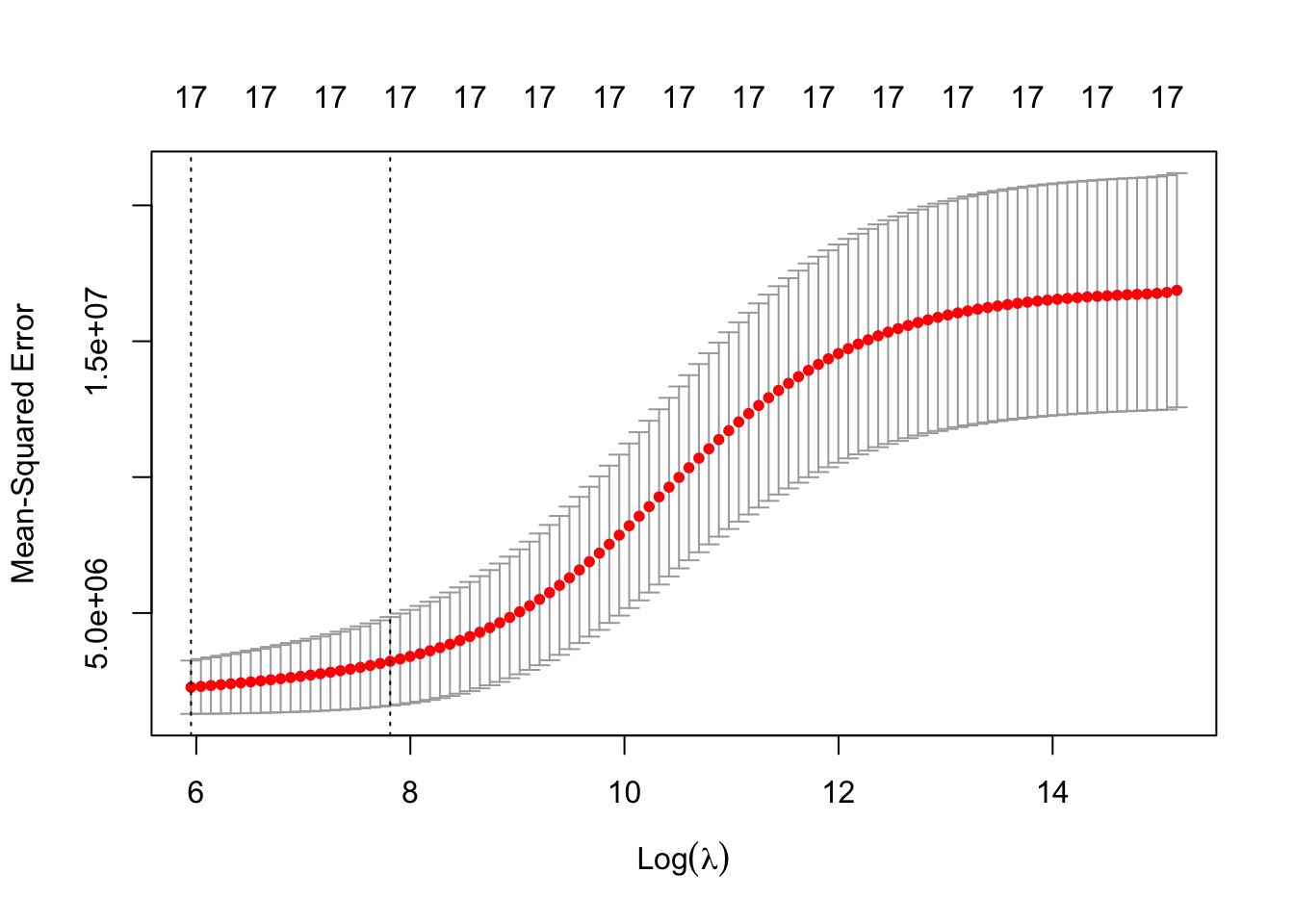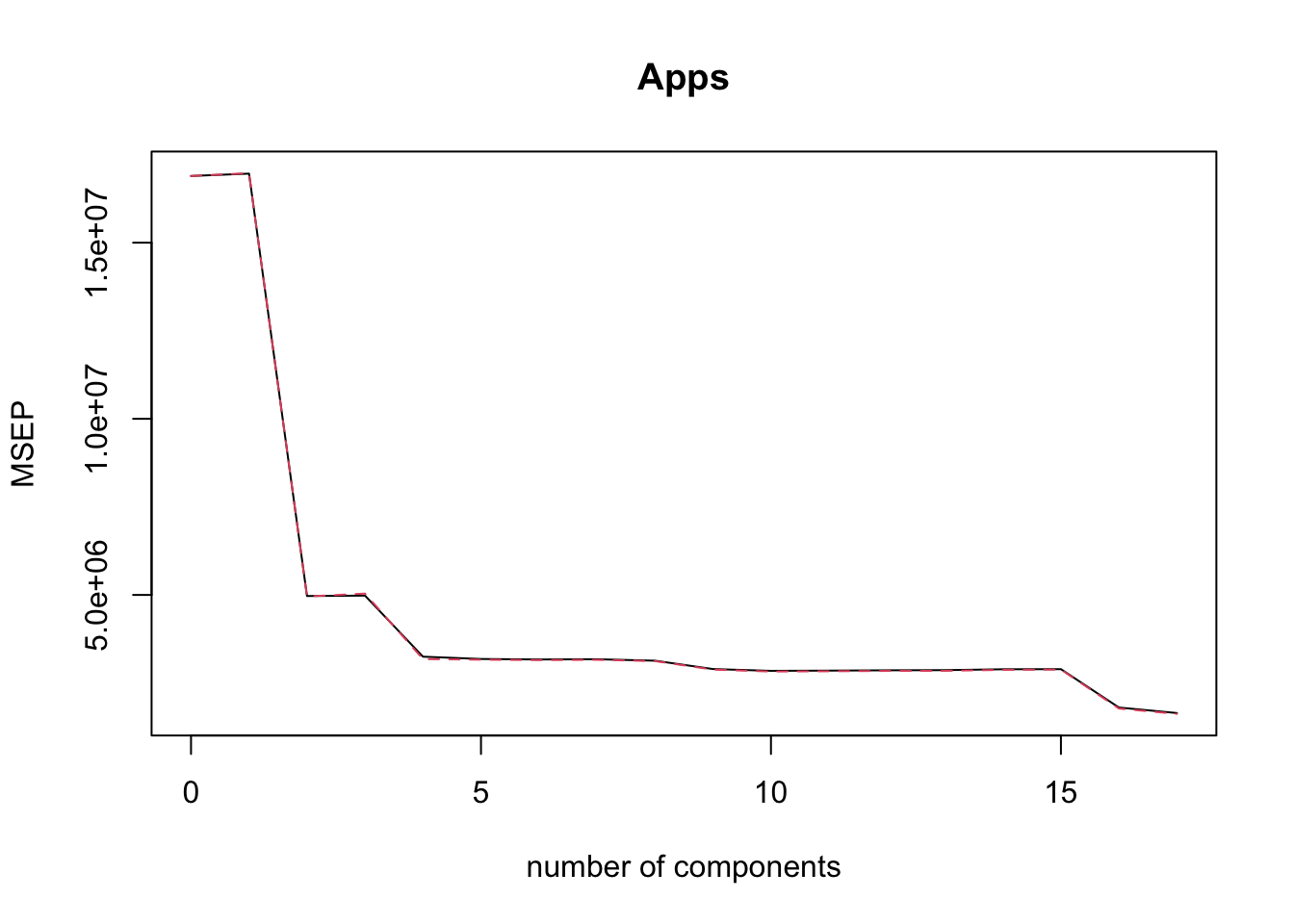ISLR Home

# Question

p263

In this exercise, we will predict the number of applications received using the other variables in the College data set.

1. Split the data set into a training set and a test set.

2. Fit a linear model using least squares on the training set, and report the test error obtained.

3. Fit a ridge regression model on the training set, with λ chosen by cross-validation. Report the test error obtained.

4. Fit a lasso model on the training set, with λ chosen by cross-validation. Report the test error obtained, along with the num- ber of non-zero coefficient estimates.

5. Fit a PCR model on the training set, with M chosen by cross-validation. Report the test error obtained, along with the value of M selected by cross-validation.

6. Fit a PLS model on the training set, with M chosen by cross-validation. Report the test error obtained, along with the value of M selected by cross-validation.

7. Comment on the results obtained. How accurately can we predict the number of college applications received? Is there much difference among the test errors resulting from these five ap- proaches?

``library(ISLR)``

# 9a

``````set.seed(11)

sum(is.na(College))``````
``##  0``
``````training <- sample(1:nrow(College), nrow(College)*.7, replace = F)
dim(College)``````
``##  777  18``
``````train <- College[training,]
test <- College[-training,]``````

# 9b

``````lm.fit = lm(Apps ~ ., data = train)

lm.predict <- predict(lm.fit, test)

mse.lm <- mean((lm.predict - test\$Apps)^2)``````

# 9c

``library(glmnet)``
``## Loading required package: Matrix``
``## Loaded glmnet 4.0-2``
``````x <- model.matrix(Apps ~ . , data = College )[,-1]
y <- College\$Apps

grid = 10 ^ seq(4, -2, length=100)

ridge.model <- glmnet(x[training,], y[training], alpha = 0, lambda = grid, thresh = 1e-12)

# WARNING: Never forget , in x[training,]
cv.out <- cv.glmnet(x[training,], y[training], alpha=0)

plot(cv.out)````````````best_lambda <- cv.out\$lambda.min
#rm(best_lamba)
ridge.pred <- predict(ridge.model, s=best_lambda, newx = x[-training,])

mse.ridge <- mean((ridge.pred - test\$Apps)^2)

mse.lm - mse.ridge``````
``##  127822.9``
``mse.lm; mse.ridge``
``##  680349.5``
``##  552526.6``

# 9d LASSO

``````lasso.model <- glmnet(x[training,], y[training], alpha = 1, lambda = grid, thresh = 1e-12)
lasso.out <- cv.glmnet(x[training,], y[training], alpha=1)
best_lambda <- lasso.out\$lambda.min

lasso.pred <- predict(lasso.model, s=best_lambda, newx = x[-training,])

mse.lasso <- mean((lasso.pred - test\$Apps)^2)
mse.lasso``````
``##  659486.5``
``mse.lm; mse.ridge; mse.lasso``
``##  680349.5``
``##  552526.6``
``##  659486.5``
``````lasso.model <- glmnet(x[training,], y[training], alpha = 1, lambda = best_lambda)
predict(lasso.model, s=best_lambda, type="coefficients")``````
``````## 18 x 1 sparse Matrix of class "dgCMatrix"
##                         1
## (Intercept) -344.69531490
## PrivateYes  -533.31988506
## Accept         1.66222553
## Enroll        -1.21722735
## Top10perc     61.57980417
## Top25perc    -20.65004529
## Outstate      -0.09509759
## Room.Board     0.13347799
## Books         -0.17312171
## Personal       0.14288779
## PhD           -9.33816497
## Terminal      -0.78991052
## S.F.Ratio     12.97049850
## perc.alumni   -1.13852874
## Expend         0.07478834

# 9e

``library(pls)``
``````##
## Attaching package: 'pls'``````
``````## The following object is masked from 'package:stats':
##
``````#set.seed(1)

pcr.fit = pcr(Apps ~ ., data = College, subset = training, scale = TRUE, validation = "CV")
summary(pcr.fit)``````
``````## Data:    X dimension: 543 17
##  Y dimension: 543 1
## Fit method: svdpc
## Number of components considered: 17
##
## VALIDATION: RMSEP
## Cross-validated using 10 random segments.
##        (Intercept)  1 comps  2 comps  3 comps  4 comps  5 comps  6 comps
## CV            4110     4118     2229     2232     1802     1784     1780
## adjCV         4110     4120     2227     2245     1783     1778     1775
##        7 comps  8 comps  9 comps  10 comps  11 comps  12 comps  13 comps
## CV        1782     1770     1702      1687      1689      1691      1693
## adjCV     1777     1767     1697      1681      1683      1686      1687
##        14 comps  15 comps  16 comps  17 comps
## CV         1700      1701      1343      1283
## adjCV      1695      1697      1332      1274
##
## TRAINING: % variance explained
##       1 comps  2 comps  3 comps  4 comps  5 comps  6 comps  7 comps  8 comps
## X      32.292    58.20    64.43    70.32    75.74    80.78    84.32    87.54
## Apps    1.699    71.48    71.50    82.31    82.44    82.67    82.80    83.11
##       9 comps  10 comps  11 comps  12 comps  13 comps  14 comps  15 comps
## X       90.44     92.81     95.00     96.87     97.91     98.78     99.39
## Apps    84.42     84.89     84.89     84.90     85.10     85.13     88.05
##       16 comps  17 comps
## X        99.85    100.00
## Apps     91.97     92.49``````
``validationplot(pcr.fit, val.type = "MSEP")````````pcr.pred <- predict(pcr.fit, x[-training,], ncomp=5)

mse.pcr <- mean((pcr.pred - test\$Apps)^2)
mse.pcr``````
``##  1664092``
``mse.lm; mse.ridge; mse.lasso; mse.pcr``
``##  680349.5``
``##  552526.6``
``##  659486.5``
``##  1664092``

# 9f PLS

``````pls.fit = plsr(Apps ~ ., data = College, subset = training, scale = TRUE, validation = "CV")
summary(pls.fit)``````
``````## Data:    X dimension: 543 17
##  Y dimension: 543 1
## Fit method: kernelpls
## Number of components considered: 17
##
## VALIDATION: RMSEP
## Cross-validated using 10 random segments.
##        (Intercept)  1 comps  2 comps  3 comps  4 comps  5 comps  6 comps
## CV            4110     2041     1741     1632     1539     1346     1289
## adjCV         4110     2037     1737     1625     1523     1326     1280
##        7 comps  8 comps  9 comps  10 comps  11 comps  12 comps  13 comps
## CV        1269     1258     1255      1253      1250      1247      1246
## adjCV     1260     1250     1248      1245      1243      1240      1239
##        14 comps  15 comps  16 comps  17 comps
## CV         1246      1247      1247      1247
## adjCV      1239      1240      1240      1240
##
## TRAINING: % variance explained
##       1 comps  2 comps  3 comps  4 comps  5 comps  6 comps  7 comps  8 comps
## X       25.97    39.56    63.72    66.17    67.98    72.26    75.16    78.48
## Apps    76.68    84.17    86.39    89.71    91.97    92.18    92.28    92.35
##       9 comps  10 comps  11 comps  12 comps  13 comps  14 comps  15 comps
## X       82.12     84.83     87.71     91.05     92.41     94.94     96.90
## Apps    92.40     92.43     92.46     92.48     92.49     92.49     92.49
##       16 comps  17 comps
## X        97.89    100.00
## Apps     92.49     92.49``````
``validationplot(pls.fit, val.type = "MSEP")``